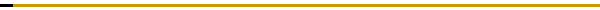# ICNAAM 20108th International Conference of Numerical Analysis and Applied MathematicsTopics to be covered include (but are not limited to): All the research areas of Numerical Analysis and Computational Mathematics (Numerical ODEs, Numerical PDEs (inc. BVPs), Scientific Computing and Algorithms, Stochastic Differential Equations, Approximation, Numerical  Linear Algebra, Numerical Integral Equations, Error Analysis and Interval Analysis, Difference Equations and Recurrence Relations, Numerical problems in Dynamical Systems, Applications to the Sciences (Computational Physics, Computational Statistics, Computational Chemistry, Computational Engineering etc.), Differential Algebraic Equations, Numerical methods in Fourier analysis etc) All the research areas of Applied and Industrial Mathematics (Mathematical Physics, Mathematical Chemistry, Mathematical Biology and Mathematical Medicine, Optimization and Operational Research, Theoretical Mechanics, Discrete Applied  Mathematics, Statistics, Probability, Dynamical Systems, Algorithms, Experimental Mathematics, Theoretical Computer Science, Applied Analysis,  Mathematical Modelling (including but not limited to  mathematical modelling of engineering and environmental processes, manufacturing, and industrial  systems, heat transfer, fluid mechanics, CFD, and transport phenomena; solid mechanics and mechanics of metals; electromagnets and MHD; reliability modelling and system optimization; decision sciences in an industrial and manufacturing context; civil engineering systems and structures; mineral and energy resources; relevant software engineering issues associated with CAD and CAE; and materials and metallurgical engineering, mathematical modelling of  social, behavioral and other sciences), Decomposition and Reconstruction Algorithms, Subdivision Algorithms, Continuous and Discrete Wavelet Transform, Time-frequency Localization, Phase-Space Analysis, Subband Coding, Image Compression, Real-Time Filtering, Radar and Sonar Applications, Transient Analysis, Medical Imaging, Multigrid Methods, Frames, Bifurcation and Singularity Theory, Deterministic Chaos and Fractals, Soliton and Coherent Phenomena, Formation of Pattern, Evolution, Complexity Theory and Neural Networks, Analytical Approaches and Simulations for more Accurate Descriptions, Predictions, Experimental Observations and Applications of Nonlinear Phenomena in Science and Engineering, Theoretical and Applied aspects of Computational Geometry, Control Theory and Automation, Fuzzy Sets and Systems and Fuzzy Logic, Applied Algebra, Quality Theory of Differential Equations, Neural Networks , etc.)